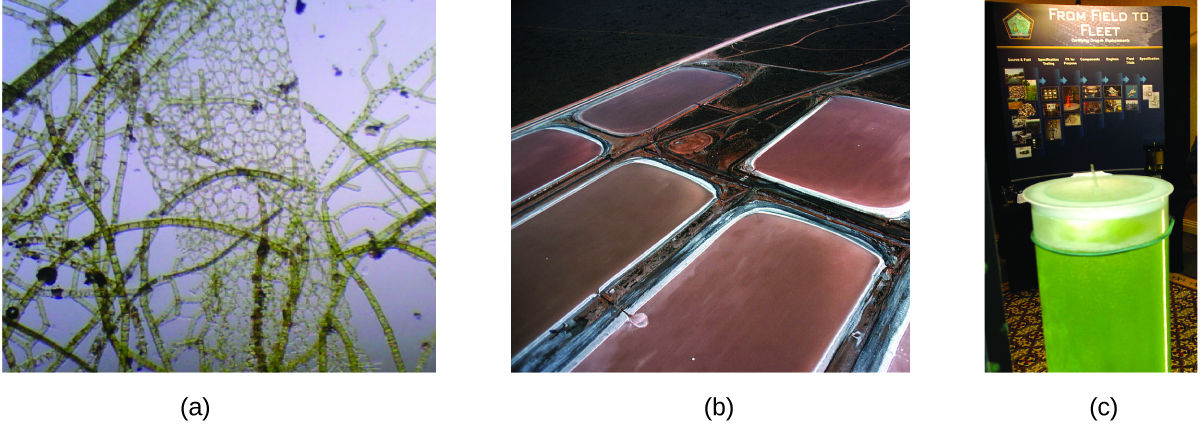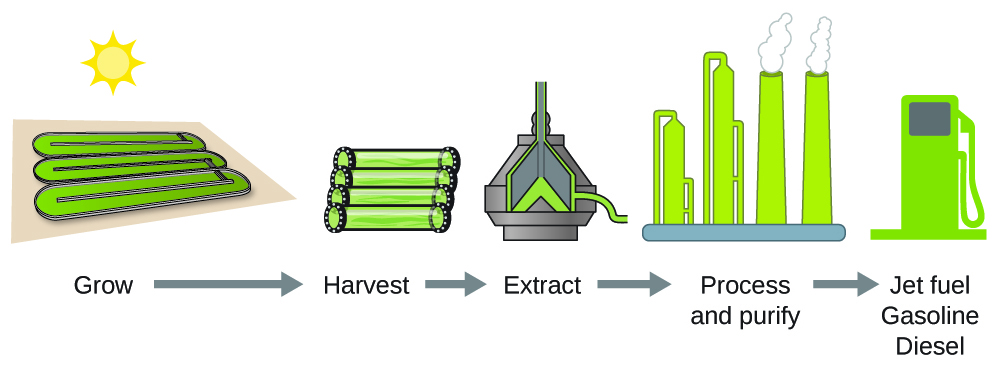# 5.3 Enthalpy  (Page 5/25)

 Page 5 / 25

## Using enthalpy of combustion

As [link] suggests, the combustion of gasoline is a highly exothermic process. Let us determine the approximate amount of heat produced by burning 1.00 L of gasoline, assuming the enthalpy of combustion of gasoline is the same as that of isooctane, a common component of gasoline. The density of isooctane is 0.692 g/mL.The combustion of gasoline is very exothermic. (credit: modification of work by “AlexEagle”/Flickr)

## Solution

Starting with a known amount (1.00 L of isooctane), we can perform conversions between units until we arrive at the desired amount of heat or energy. The enthalpy of combustion of isooctane provides one of the necessary conversions. [link] gives this value as −5460 kJ per 1 mole of isooctane (C 8 H 18 ).

Using these data,

$1.00\phantom{\rule{0.2em}{0ex}}\overline{)\text{L}\phantom{\rule{0.2em}{0ex}}{\text{C}}_{8}{\text{H}}_{18}}\phantom{\rule{0.2em}{0ex}}×\phantom{\rule{0.2em}{0ex}}\frac{1000\phantom{\rule{0.2em}{0ex}}\overline{)\text{mL}\phantom{\rule{0.2em}{0ex}}{\text{C}}_{8}{\text{H}}_{18}}}{1\phantom{\rule{0.2em}{0ex}}\overline{)\text{L}\phantom{\rule{0.2em}{0ex}}{\text{C}}_{8}{\text{H}}_{18}}}\phantom{\rule{0.2em}{0ex}}×\phantom{\rule{0.2em}{0ex}}\frac{0.692\phantom{\rule{0.2em}{0ex}}\overline{)\text{g}\phantom{\rule{0.2em}{0ex}}{\text{C}}_{8}{\text{H}}_{18}}}{1\phantom{\rule{0.2em}{0ex}}\overline{)\text{mL}\phantom{\rule{0.2em}{0ex}}{\text{C}}_{8}{\text{H}}_{18}}}\phantom{\rule{0.2em}{0ex}}×\phantom{\rule{0.2em}{0ex}}\frac{1\phantom{\rule{0.2em}{0ex}}\overline{)\text{mol}\phantom{\rule{0.2em}{0ex}}{\text{C}}_{8}{\text{H}}_{18}}}{114\phantom{\rule{0.2em}{0ex}}\overline{)\text{g}\phantom{\rule{0.2em}{0ex}}{\text{C}}_{8}{\text{H}}_{18}}}\phantom{\rule{0.2em}{0ex}}×\phantom{\rule{0.2em}{0ex}}\frac{-5460\phantom{\rule{0.2em}{0ex}}\text{kJ}}{1\phantom{\rule{0.2em}{0ex}}\overline{)\text{mol}\phantom{\rule{0.2em}{0ex}}{\text{C}}_{8}{\text{H}}_{18}}}\phantom{\rule{0.2em}{0ex}}=-3.31\phantom{\rule{0.2em}{0ex}}×\phantom{\rule{0.2em}{0ex}}{10}^{4}\text{kJ}$

The combustion of 1.00 L of isooctane produces 33,100 kJ of heat. (This amount of energy is enough to melt 99.2 kg, or about 218 lbs, of ice.)

Note: If you do this calculation one step at a time, you would find:

$\begin{array}{l}\\ 1.00\phantom{\rule{0.2em}{0ex}}\text{L}\phantom{\rule{0.2em}{0ex}}{\text{C}}_{8}{\text{H}}_{18}\phantom{\rule{0.2em}{0ex}}⟶\phantom{\rule{0.2em}{0ex}}1.00\phantom{\rule{0.2em}{0ex}}×\phantom{\rule{0.2em}{0ex}}{10}^{3}\phantom{\rule{0.2em}{0ex}}\text{mL}\phantom{\rule{0.2em}{0ex}}{\text{C}}_{8}{\text{H}}_{18}\\ 1.00\phantom{\rule{0.2em}{0ex}}×\phantom{\rule{0.2em}{0ex}}{10}^{3}\phantom{\rule{0.2em}{0ex}}\text{mL}\phantom{\rule{0.2em}{0ex}}{\text{C}}_{8}{\text{H}}_{18}\phantom{\rule{0.2em}{0ex}}⟶\phantom{\rule{0.2em}{0ex}}692\phantom{\rule{0.2em}{0ex}}\text{g}\phantom{\rule{0.2em}{0ex}}{\text{C}}_{8}{\text{H}}_{18}\\ 692\phantom{\rule{0.2em}{0ex}}\text{g}\phantom{\rule{0.2em}{0ex}}{\text{C}}_{8}{\text{H}}_{18}\phantom{\rule{0.2em}{0ex}}⟶\phantom{\rule{0.2em}{0ex}}6.07\phantom{\rule{0.2em}{0ex}}\text{mol}\phantom{\rule{0.2em}{0ex}}{\text{C}}_{8}{\text{H}}_{18}\\ 692\phantom{\rule{0.2em}{0ex}}\text{g}\phantom{\rule{0.2em}{0ex}}{\text{C}}_{8}{\text{H}}_{18}\phantom{\rule{0.2em}{0ex}}⟶\phantom{\rule{0.2em}{0ex}}-3.31\phantom{\rule{0.2em}{0ex}}×\phantom{\rule{0.2em}{0ex}}{10}^{4}\text{kJ}\end{array}$

How much heat is produced by the combustion of 125 g of acetylene?

6.25 $×$ 10 3 kJ

## Emerging algae-based energy technologies (biofuels)

As reserves of fossil fuels diminish and become more costly to extract, the search is ongoing for replacement fuel sources for the future. Among the most promising biofuels are those derived from algae ( [link] ). The species of algae used are nontoxic, biodegradable, and among the world’s fastest growing organisms. About 50% of algal weight is oil, which can be readily converted into fuel such as biodiesel. Algae can yield 26,000 gallons of biofuel per hectare—much more energy per acre than other crops. Some strains of algae can flourish in brackish water that is not usable for growing other crops. Algae can produce biodiesel, biogasoline, ethanol, butanol, methane, and even jet fuel.(a) Tiny algal organisms can be (b) grown in large quantities and eventually (c) turned into a useful fuel such as biodiesel. (credit a: modification of work by Micah Sittig; credit b: modification of work by Robert Kerton; credit c: modification of work by John F. Williams)

According to the US Department of Energy, only 39,000 square kilometers (about 0.4% of the land mass of the US or less than $\frac{1}{7}$ of the area used to grow corn) can produce enough algal fuel to replace all the petroleum-based fuel used in the US. The cost of algal fuels is becoming more competitive—for instance, the US Air Force is producing jet fuel from algae at a total cost of under \$5 per gallon. For more on algal fuel, see http://www.theguardian.com/environment/2010/feb/13/algae-solve-pentagon-fuel-problem. The process used to produce algal fuel is as follows: grow the algae (which use sunlight as their energy source and CO 2 as a raw material); harvest the algae; extract the fuel compounds (or precursor compounds); process as necessary (e.g., perform a transesterification reaction to make biodiesel); purify; and distribute ( [link] ).Algae convert sunlight and carbon dioxide into oil that is harvested, extracted, purified, and transformed into a variety of renewable fuels.

mention some examples of ester
do you mean ether?
Megan
what do converging lines on a mass Spectra represent
would I do to help me know this topic ?
Bulus
oi
Amargo
what the physic?
who is albert heistein?
Bassidi
similarities between elements in the same group and period
what is the ratio of hydrogen to oxulygen in carbohydrates
bunubyyvyhinuvgtvbjnjnygtcrc
yvcrzezalakhhehuzhbshsunakakoaak
what is poh and ph
please what is the chemical configuration of sodium
Sharon
2.8.1
david
1s²2s²2p⁶3s¹
Haile
2, 6, 2, 1
Salman
1s2, 2s2, 2px2, 2py2, 2pz2, 3s1
Justice
1s2,2s2,2py2,2
Maryify
1s2,2s2,2p6,
Francis
1s2,2s2,2px2,2py2,2pz2,3s1
Nnyila
what is criteria purity
cathode is a negative ion why is it that u said is negative
cathode is a negative electrode while cation is a positive ion. cation move towards cathode plate.
king
CH3COOH +NaOH ,complete the equation
compare and contrast the electrical conductivity of HCl and CH3cooH
The must be in dissolved in water (aqueous). Electrical conductivity is measured in Siemens (s). HCl (aq) has higher conductivity, as it fully ionises (small portion of CH3COOH (aq) ionises) when dissolved in water. Thus, more free ions to carry charge.
Abdelkarim
HCl being an strong acid will fully ionize in water thus producing more mobile ions for electrical conduction than the carboxylic acid
Valentine
differiante between a weak and a strong acid
david
how can I tell when an acid is weak or Strong
Amarachi
an aqueous solution of copper sulphate was electrolysed between graphite electrodes. state what was observed at the cathode
write the equation for the reaction that took place at the anode
Bakanya
what is enthalpy of combustion
Bakanya
Enthalpy change of combustion: It is the enthalpy change when 1 mole of substance is combusted with excess oxygen under standard conditions. Elements are in their standard states. Conditions: pressure = 1 atm Temperature =25°C
Abdelkarim
Observation at Cathode: Cu metal deposit (pink/red solid).
Abdelkarim
Equation at Anode: (SO4)^2- + 4H^+ + 2e^- __> SO2 + 2H2O
Abdelkarim
Equation : CuSO4 -> Cu^2+ + SO4^2- equation at katode: 2Cu^2+ + 4e -> 2Cu equation at anode: 2H2O -> 4H+ + O2 +4e at the anode which reacts is water because SO4 ^ 2- cannot be electrolyzed in the anode
Niken
what is the electrolysis of sulphuric acid
why is electrolysis difficult using solid lead chloride
Bakanya
what is heat formation
what are atoms
this are small substances that form together and complained one
Belvine
okay gud
Bol
what's covalent bonding
Covalent bonds are characterized by the sharing of electrons between two or more atoms. These bonds mostly occur between nonmetals or between two of the same (or similar) elements.
Haile
covalent bonding is the mutual sharing of electrons between two element in a molecule, usually it involves non metals as they are less ionic and more electronegative than metals( ionic). and these bonds have high enthalpy of formation. and are strong bonds than most of the bond.
Chiranjeev
covalent bonding involves both nonmetals where there is complete sharing of electrons on the outermost energy level
david
I don't understand
BelvineByByBy Madison ChristianBy Brooke DelaneyBy Janet ForresterBy Carly AllenBy David CoreyBy John GabrieliBy OpenStaxBy OpenStaxBy John GabrieliBy OpenStax